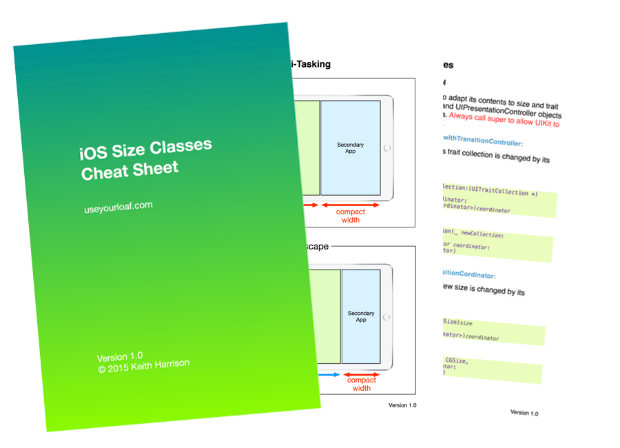# Character Properties in Swift 5

The release of Swift 5 is coming with Xcode 10.2 and brings some updates to the String API in the standard library. Here’s a look at the new character properties that allow you to test for a number of useful Unicode properties.

I have updated my Swift String Cheat Sheet and playground with the changes. You can find them here:

### New Character Properties

The Unicode standard defines a number of character properties such as whether the character is upper or lower case, whitespace, punctuation, etc. Writing these tests yourself in a way that is reliable for all text encodings and alphabets is non-trivial.

Swift Evolution proposal SE-0221 Character Properties has been implemented in Swift 5 to add a number of convenient properties to `Character` based on the Unicode standards:

#### Testing For ASCII

Test if a character is ASCII (note that these properties operate on characters not strings):

``````let a = "A" as Character
let pi = "π" as Character
a.isASCII               // true
pi.isASCII              // false
``````

The `asciiValue` property is an optional integer that returns the ASCII value (or nil if the character is not ASCII):

``````a.asciiValue            // Int? (65)
pi.asciiValue           // nil
``````

#### Testing For Whitespace and New Lines

The `isWhitespace` property tests for spaces and other separator characters:

``````let tab = "\t" as Character
tab.isWhitespace        // true
``````

A new line character is also classed as whitespace. The `isNewline` property tests more specifically for it (and other line separators):

``````let newline = "\n" as Character
newline.isWhitespace    // true
newline.isNewline       // true
``````

#### Testing For Numbers

Test for numbers and whole numbers:

``````let five = "5" as Character
let half = "½" as Character
five.isNumber           // true
half.isNumber           // true
``````

If the character is a whole number then `wholeNumberValue` gives you the numeric value:

``````five.isWholeNumber      // true
five.wholeNumberValue   // Int? (5)
half.isWholeNumber      // false
half.wholeNumberValue   // nil
``````

This also works for hexadecimal characters (upper or lower case):

``````let a = "A" as Character
a.isHexDigit            // true
a.hexDigitValue         // Int? (10)
``````

#### Testing For Letters

Does the character represent an alphabetic letter:

``````a.isLetter              // true
pi.isLetter             // true (Greek alphabet)

let scream = "😱" as Character
scream.isLetter         // false
``````

#### Testing For Symbols

Test if a character is a symbol:

``````let smiley = "😀" as Character
smiley.isSymbol         // true
smiley.isLetter         // false

let plus = "+" as Character
plus.isSymbol           // true
plus.isLetter           // false
``````

Test for a math symbol:

``````plus.isMathSymbol       // true
smiley.isMathSymbol     // false
``````

Test for a currency symbol:

``````let dollar = "\$" as Character
dollar.isCurrencySymbol // true
``````

#### Punctuation

To test for punctuation marks:

``````let qmark = "?" as Character
qmark.isPunctuation     // true
``````

#### Upper And Lower Case

Properties to test for case and functions to convert the case:

``````let b = "b" as Character
let z = "Z" as Character
b.isLowercase           // true
z.isUppercase           // true
``````

The functions to convert to upper or lower case return a `String` as it can result in multiple characters:

``````b.uppercased()          // B
pi.uppercased()         // Π
z.lowercased()          // z

let sharpS = "ß" as Character
sharpS.uppercased()     // SS
``````

The `isCased` property is a strange one. It tests if the character changes when converted to upper or lower case:

``````z.isCased               // true (z or Z)
b.isCased               // true (b or B)

let half = "½" as Character
half.isCased            // false (always ½)
``````